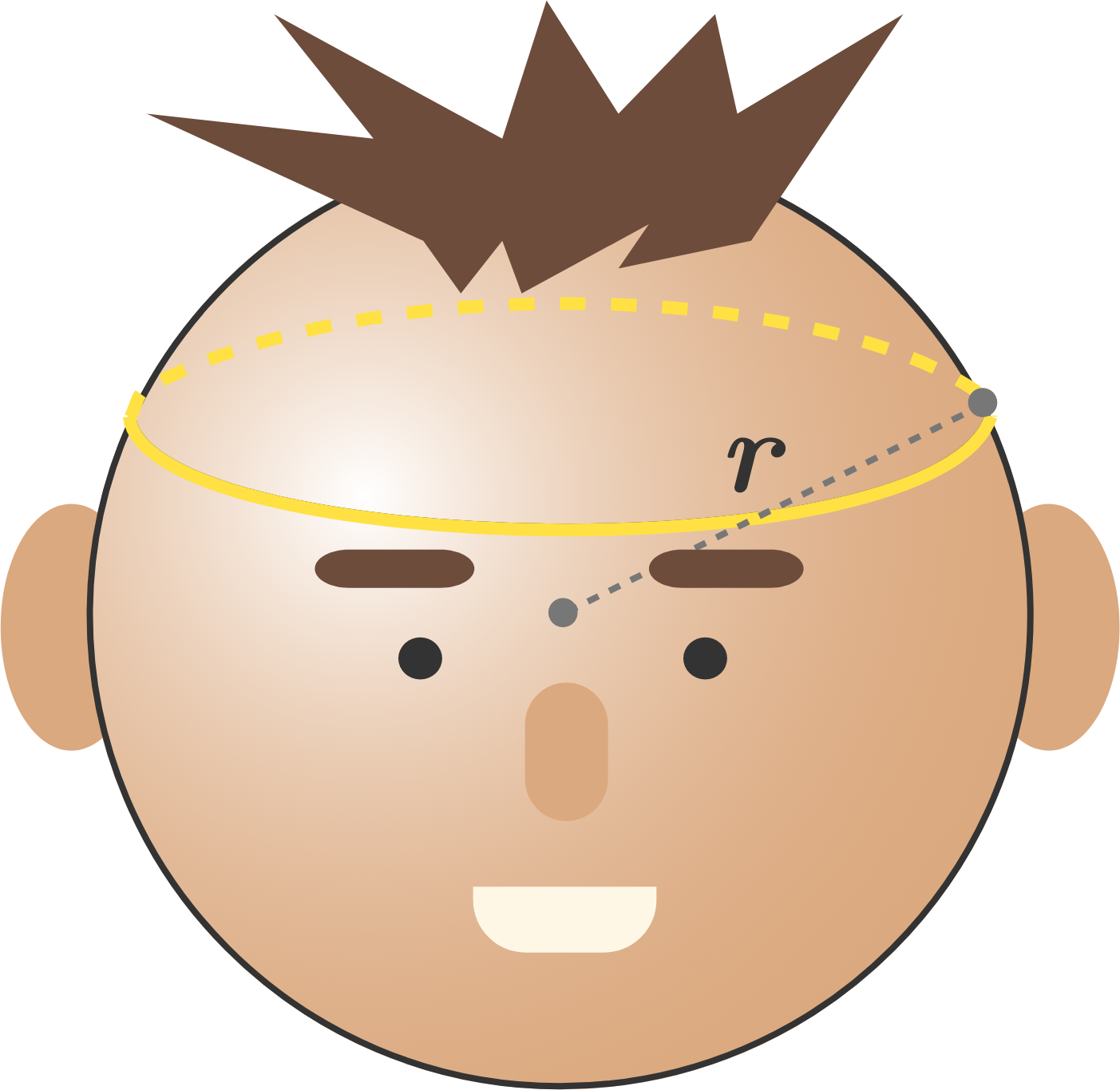# Tension for the crownThe blacksmiths of the kingdom of Mechania are wondering how much tension is holding a crown together as it rests on the head of their sovereign. The king of Mechania has a frictionless, perfectly spherical head with radius $r = 10 \text{ cm}$, and the crown is a thin cord with a length of $\ell = 37.7 \text{ cm}$ and a mass of $m = 628.32 \text{ g}.$

Compute the tension in the crown (in Newtons).

Assumptions:

• The crown has no thickness and rests perfectly level (that is, it makes a circle which is contained in a plane parallel to the horizontal).
• The gravitational constant of Mechania is $g = 10 \text{ m/s}^2.$
×